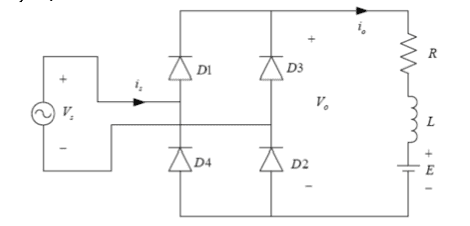# Bridge rectifier output voltage

Hi, I'm trying to figure out why the output voltage waveform of this circuit would not include a DC offset of E. My understanding is that D1 becomes forward biased when Vs > E, and so that is when it should conduct. Every waveform drawing I've seen, on the other hand, begins conduction at 0 volts.Also, what would be the difference between this circuit and one that replaces two diodes with thyristors? I've seen the latter referred to as half-controlled.

Thanks!

mfb
Mentor
I didn't analyze the circuit in detail, but don't forget the coil: At Vs close to 0 you have the fastest voltage changes -> current changes in the resistor -> the coil will have some induced voltage.

At which nodes is the output voltage taken?

256bits
Gold Member
Take the inductor out of the circuit and you have a diode clipping circuit, where the diode will not conduct until the input waveform Vs > Vd + E. You can see the if you remove the R as shown and instead add an R to the anodes of D1 and D3, to obtain the textbook diode clipping circuit, where the output is taken anode of D1 to the bottom of the circuit. Of course, you have another diode in there, D2, but that is just another 0.7v ( for silicon ).

There is no DC bias voltage in a clipping circuit in the normal sense of where the output voltage for a clipping circuit is taken. Textbooks will add in a capacitor for DC bias and show the output waveform at the appropriate connections.

Then you can add in the inductor to see how that affects the circuit.

Skeptic is correct. One has to specify the nodes to properly identify the output waveform.

Last edited: